Next: Example 6.3: Equivalent capacitance Up: Capacitance Previous: Example 6.1: Parallel plate

## Example 6.2: Dielectric filled capacitor

Question: A parallel plate capacitor has a plate area of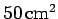and a plate separation of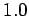cm. A potential difference of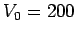V is applied across the plates with no dielectric present. The battery is then disconnected, and a piece of Bakelite (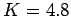) is inserted which fills the region between the plates. What is the capacitance, the charge on the plates, and the potential difference between the plates, before and after the dielectric is inserted?

Answer: Before the dielectric is inserted, the space between the plates is presumably filled with air. Since the dielectric constant of air is virtually indistinguishable from that of a vacuum, let us use the vacuum formula (108) to calculate the initial capacitance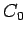. Thus,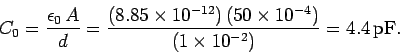After the dielectric is inserted, the capacitance increases by a factor, which in this case is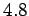, so the new capacitance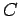is given by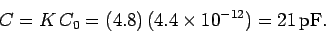Before the dielectric is inserted, the charge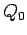on the plates is simply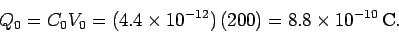After the dielectric is inserted, the chargeis exactly the same, since the capacitor is disconnected, and so the charge cannot leave the plates. Hence,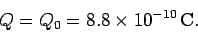The potential difference before the dielectric is inserted is given asV. The potential differenceafter the dielectric is inserted is simply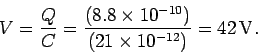Note, of course, that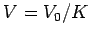.Next: Example 6.3: Equivalent capacitance Up: Capacitance Previous: Example 6.1: Parallel plate
Richard Fitzpatrick 2007-07-14